# What do those digits in your VIN/Chassis number mean?

08.08.2016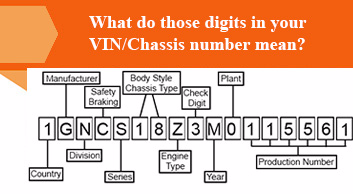Every single digit in your Vehicle identification number have a meaning and it is easy to know the details about your vehicle through those 17 digits and don't worry it is totally safe to share you chassis number with anyone.

The Vehicle Identification Number (VIN) is a unique number given to every vehicle in the world and contains information related to the vehicle and manufacturer. The VIN is made of 17 characters/digits with each one specifying a certain detail of the car.

On studying what portion of the VIN signifies what property, one should divide them into three groups.

## Group 1: First three letters

The first letters denote the World Manufacturer Identifier number.

1st Position: The first character/digit is about which region of the world your vehicle was manufactured. See the below for the character/digit and the respective region they represent.

• Africa - A, B, C, D, E, F, G, and H
• Asia - J, K, L, M, N, P, and R
• Europe - S, T, U, V, W, X, Y, and Z
• North America - 1, 2, 3, 4, 5
• Oceania - 6 and 7
• South America - 8 and 9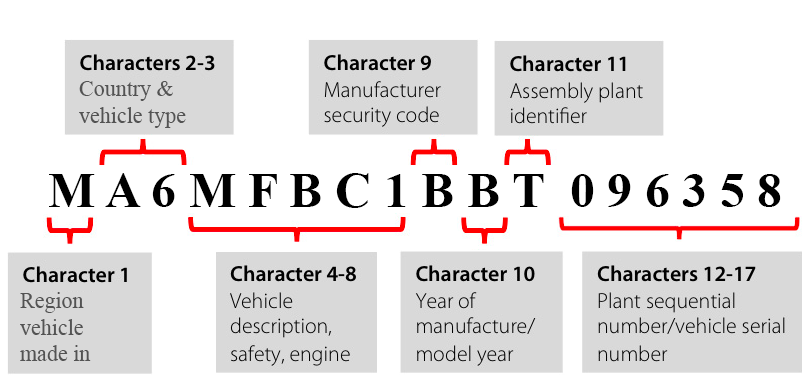2nd Position: The second character/digit in combination with the first letter will tell you in which country the automobile was made. In total, there are over 600 VIN codes for countries and manufacturers often make cars in different parts of the world.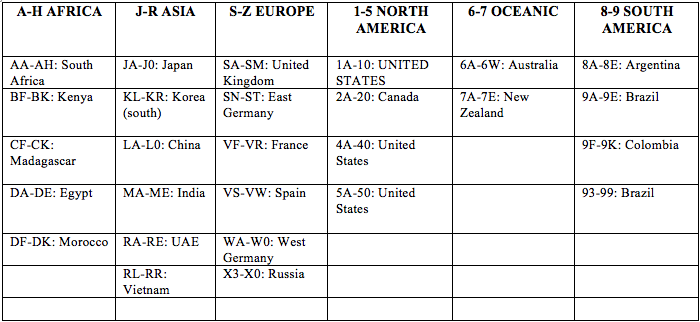3rd Position: The third character/digit is about what kind of vehicle it is classified as, whether it is a car, motorcycle, truck or bus. Each manufacturer uses different codes for this, which is given in their respective website and documentations.

## Group 2: Positions from 4 to 9

This group refers to the Vehicle Description Section. It carries information about the vehicle itself.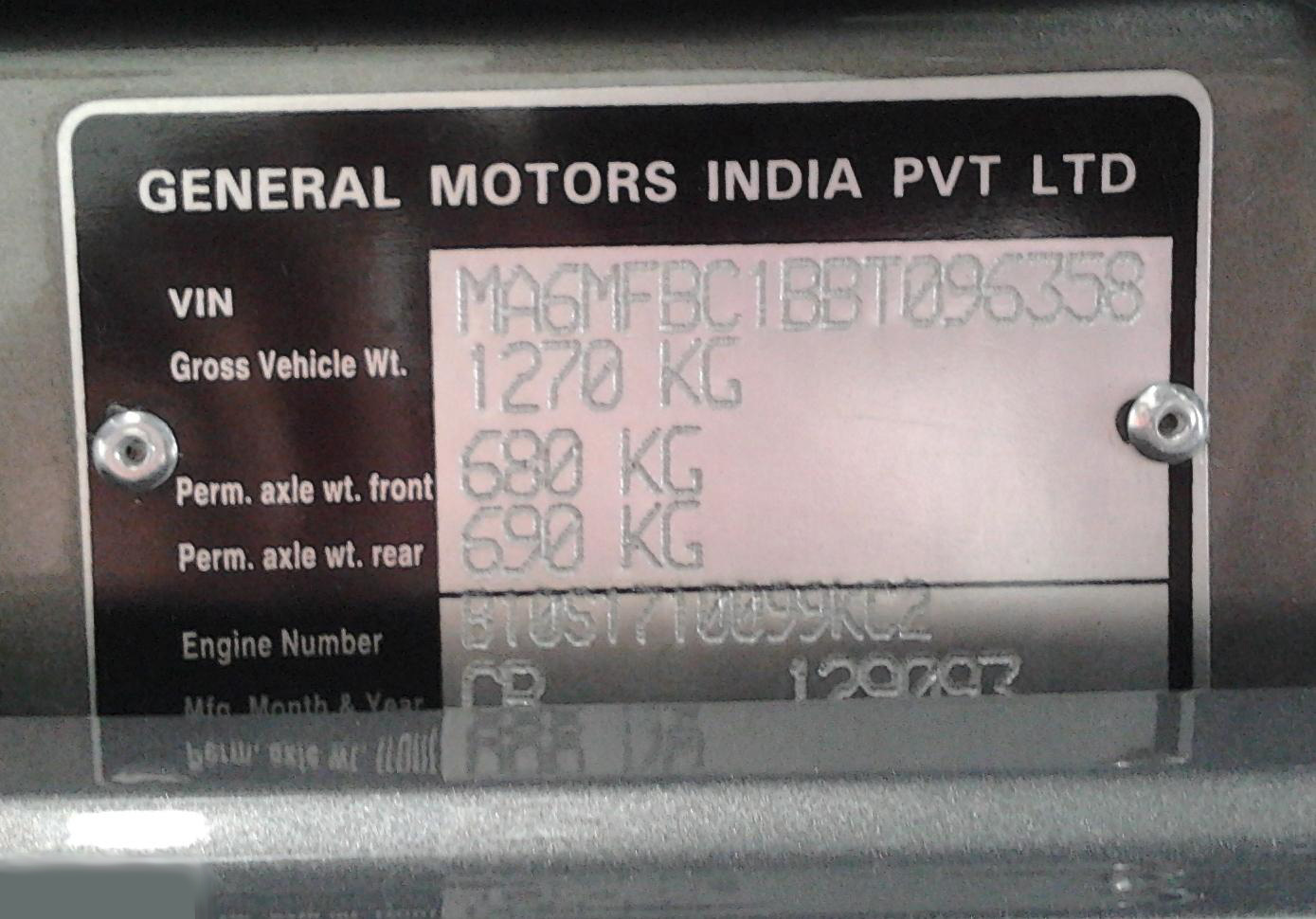4th – 8th Position – These character/digits pertain to information regarding the vehicle model, body style, and engine type. The codes vary with each automobile maker.

9th Position – This is the check digit which tells you if the VIN is correct.

Calculating Check Digit –

The check digit is placed in every vehicle’s VIN. There are two ways of checking the VIN through the check digit, first by removing the check digit and then calculating or secondly using a weight of 0 to cancel it. After calculation, the calculated value is compared to the check digit value. If the calculated value is between 0 and 9 then the check digit must exactly match the calculated value. If the calculated value comes to 10 then the check digit must be the character X. If the calculated value is different from check digit value then the VIN is wrong (this can be stated for an accuracy of 90%).

Steps for calculation -

1.              For calculation, every character is assigned a numeric value (Numeric values are taken as same) and each position of the VIN is also given a weight value.

Character Values -

A = 1, B = 2, C = 3, D = 4, E = 5, F = 6, G = 7, H = 8,

J = 1, K = 2, L = 3, M = 4, N = 5, O = 6, P = 7, R = 9,

S = 2, T = 3, U = 4, V = 5, W = 6, X = 7, Y = 8, Z = 9

Position Weightage -

1st = 8, 2nd = 7, 3rd = 6, 4th = 5, 5th = 4, 6th = 3, 7th = 2, 8th = 10,

9th = 0 (check digit), 10th = 9, 11th = 8, 12th = 7, 13th = 6, 14th = 5,

15th = 4, 16th = 3, 17th = 2

2.              Then character/digit value is multiplied with the weight value of corresponding VIN position.

3.              Then all the products are added and divided by 11.

4.              The remainder from the division is taken as the check digit.

## Group 3 – Position from 10 to 17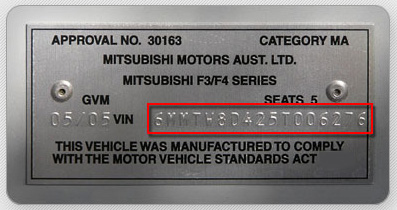10th Position – The 10th character/digit signifies the year of the car model, which may sometimes differ to the manufacturing year. Vehicle makers sometimes make cars in advance and sell them the following year. It should also be noted that once all the digits/characters from A to Z and 1 to 9 were exhausted they were again started in the same series. Therefore one should check 7th VIN position if it is a character then your vehicle was made post-2010 and if it is a number then it was prior to 2010.

11th – 17th Position – Here the manufacturer adds extra information which is unique to the vehicle. Information like which assembly plant the vehicle was manufactured at, the production sequence number and extra accessories and modifications added. The codes vary with each company and there are given in the company documentation and website.